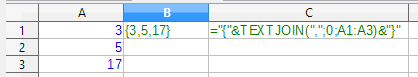# Calc - using tuples/vectors

Let’s say I have values

``````A1 = 3
A2 = 5
A3 = 17
``````

How do I get calc to display a vector containing these three values in cell B1? I.e:

``````B1= (3,5,17)
``````

And can I then just - for example -

``````C1=AVERAGE(B1)
``````

to get

``````C1 = 8.33333
``````

?

(FINAL amendment regarding the comments by the OQ:)
See this new attachment!

(Original answer based on misunderstandings due to unclear question:)
Cells can have three content types: Number, Text, Formula
Cells can have two(three) result types: Number, Text (and Error) either from direct entry or as a formula result.
There is neither a content type nor a result type like Vector or Array …
Among the results (may be direct content, too) of type Text there can also be strings determining a reference like “MySheet.A1:A3” (without the quotes) e.g. In such a case the function INDIRECT applied to the cell will return the reference itself. Another way to reference a range (vector or 2D-array) - exemplified for the above range again - is `=OFFSET(MySheet.A1;0;0;3;1)`.

With `A1:A3` in cell `B1` of the same sheet, `=AVERAGE(INDIRECT(B1))` or `=AVERAGE(OFFSET(A1;0;0;3;1))` will work as expected and return the result `=AVERAGE(A1:A3)` also would.

Not as the content of a cell but as a sub-expression in a formula you may also use the type Constant Inline Array. The precise syntax is depending on the locale and/or your options settings. An as-if-row CIL might look like `{3,5,17}` (Regard the curly brackets!) e.g. in a locale not using the comma as its decimal separator. `=AVERAGE({3,5,17})` will then return 8.33… CIL are not accepted by INDIRECT. They rarely are of much use.

(Editing with respect to the first two comments below:)
Most likely a formula like `=AVERAGE(OFFSET(INDIRECT(C1);0;0;C2;C3))` would offer all the desirable flexibility: With the address (K5 e.g.) of the cell to start with as text in C1, the number of rows to include (9 e.g.) in C2, and the number of columns (3 e.g.) to include in C3 the above formula will calculate as if it was `=AVERAGE(K5:M13)` (regarding the given example values). See attached example.

So … what you’re saying is I need to write `B1="("&A1&","&A2&","&A3&")"` and then `C1=AVERAGE(A1:A3)`. You wouldn’t happen to know how to write a macro to which I can pass a variable number of arguments (single cells or cell ranges) and that will construct me the obnoxious `="("&X1&","&Y4002&","&Z19&"," & ... & ")"` formula?

Sorry. I didn’t write (intentionally) anything to that effect. My suppopsed entry into B1 was `\$A1:\$A3`. This to later apply the INDIRECT function on B1.
Now I wonder in what sense you are using the term “variable number of arguments”. If it’s about “parameters” I would need to know more detail.
If you want to handle a contiguous cellrange with a variable number of rows and/or columns I think I already told you that OFFSET is the appropriate function.

why the hell do you want to work contrary to the way calc does `???`

Woul you mind to explain?
I don’t think my posts here are of kind “why the hell”. @DiesNuts on the other hand obviously is still rather inexperienced. May be he should read a bit in the Calc guide.
Needs to get parametriesed ranges are the reason for the implementation of OFFSET and of INDIRECT as well).

@Lupp thank you, I am aware of the OFFSET and the INDIRECT.
What I NEED, though, is an agreeable representation so I can export the table and include it into a latex document. So as that’s not supported, I’m looking for a macro that will produce that for me. E.g. let’s call that macro `vec`. so `=vec(A1)` should produce `="("&A1&")"`, `=vec(A1:A3)` should produce `="("&A1&","&A2&","&A3&")"`, `=`vec(A1,X5:X99,Z3)`should result in`="("&A1&","&“X5”&","&X6&…&x99&","&Z3&")"``

it’s not a particularly hard macro, I just have no idea how to code it in calc.

Perhaps TEXTJOIN() can help, but the result it’s a text.That looks like what I’m looking for … but my libre office calc doesn’t know any `TEXTJOIN` function.

The TEXTJOIN function is implemented (preliminary) in LibO V5.2.0 and higher. See also this bug. If you have problems due to the bug, please come back and tell. I coded a version in BASIC without the bug and slightly more powerful.
(If the subject was “Concatenating contents from arbitrary ranges” I knew how to help from the beginning.)

@Lupp I would appreciate it if you could share that BASIC version because I cannot upgrade to 5.2 (tried, it got me `W: Failed to fetch http://ppa.launchpad.net/libreoffice/libreoffice-5-2/ubuntu/dists/wily/main/binary-amd64/Packages 404 Not Found`

`W: Failed to fetch http://ppa.launchpad.net/libreoffice/libreoffice-5-2/ubuntu/dists/wily/main/binary-i386/Packages 404 Not Found`

@DiesNuts: This is not about your problem with installing V5.2.x.y on Ubuntu (which should be solved, of course).
See demo attached to my answer and containing code and examples. As this should be the final version I inserted the amendment at the beginning of the answer.